Courses

# Test: Highway Materials & Testing- 2

## 10 Questions MCQ Test Transportation Engineering | Test: Highway Materials & Testing- 2

Description
This mock test of Test: Highway Materials & Testing- 2 for Civil Engineering (CE) helps you for every Civil Engineering (CE) entrance exam. This contains 10 Multiple Choice Questions for Civil Engineering (CE) Test: Highway Materials & Testing- 2 (mcq) to study with solutions a complete question bank. The solved questions answers in this Test: Highway Materials & Testing- 2 quiz give you a good mix of easy questions and tough questions. Civil Engineering (CE) students definitely take this Test: Highway Materials & Testing- 2 exercise for a better result in the exam. You can find other Test: Highway Materials & Testing- 2 extra questions, long questions & short questions for Civil Engineering (CE) on EduRev as well by searching above.
QUESTION: 1

### What is the weight of the charge used in the Los Angeles abrasion test?

Solution:

As per IS 2386 part 4, the steel balls are used as the charge for the test. They are of 48 mm diameter and each ball weighs around 416.67 g. Depending on the grading used for the test, the number of balls used changes.

QUESTION: 2

### The following particulars are obtained after conducting the test for angularity number: Volume of cylinder = 3000 g Specific gravity = 2.68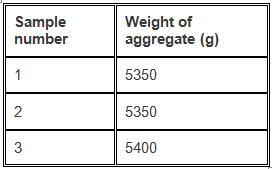What is the angularity number for the above sample?

Solution:

Angularity number is obtained using the formula,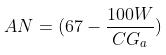Where W represents the mean weight of aggregate (g),
C represents the volume of the cylinder (g) and,
Ga represents the specific gravity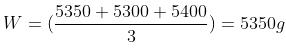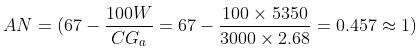The angularity number is represented as a whole number and is rounded off to nearest one.

QUESTION: 3

### There are six types of tests to conduct the adhesion test on aggregates.

Solution:

The tests to determine the stripping value can be classified into six types. They are static immersion test, dynamic immersion test, chemical immersion test, immersion mechanical test, immersion trafficking test and coating test.

QUESTION: 4

The result for soundness test is expressed in terms of ______

Solution:

To conduct the soundness test, the aggregates are subjected to solutions of sodium sulphate or magnesium sulphate as per IS 2386. After cycles of immersion and drying, the loss in weight of aggregate is noted down in percentage and that gives the soundness result.

QUESTION: 5

The combined index is obtained by ______ the flakiness and elongation index.

Solution:

Combined index is obtained as the sum of both flakiness and elongation index. The sample is first subjected to flakiness test, then the non-flaky particles are subjected to the elongation test. Their indices are found out and added together.

QUESTION: 6

What is used as a charge in the Dorry abrasion test?

Solution:

Dorry abrasion test was used way back and is not used these days. The charge used for the test was sand. It was let into the machine to cause abrasive action. Crushed gravel cannot be used as it is the same material and it would cause attrition, not abrasion.

QUESTION: 7

After the abrasion test, the sample is passed through which sieve?

Solution:

The sample after removing from the abrasion machine is passed through 1.7 mm sieve and the weight is noted down. 2.36 mm is used in the impact and crushing test on aggregates.

QUESTION: 8

The aggregate impact test was conducted on a sample and the following readings were taken:
Weight of sample taken () = 350 g
Weight of sample passing 2.36 mm sieve () = 92 g
What will be the aggregate impact value for the sample?

Solution:

The aggregate impact value is obtained as the ratio of the weight of sample passing 2.36 mm sieve to the weight of the sample taken for the test. For the readings in the question, the aggregate impact value can be found out as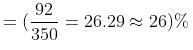and is expressed as a whole number in percentage.

QUESTION: 9

Which of the below test – procedure pairs are matched correctly?

Solution:

As per IS 2386 part 3, in the impact test, the sample is filled in three layers and given 25 tamping each. As per IS 2386 part 1, the sample is filled in three layers and tamped 100 times each for the angularity number. As per IS 2386 part 3, in the bulk density test, the sample is filled in three layers and given 25 tamping each. As per IS 2386 part4, the sample for crushing test is filled in three layers and tamped 25 times each.

QUESTION: 10

The polished stone value of an aggregate is reported as the ______ of the two values of ______

Solution:

There are two stages conducted for this test. The sample is polished in the first stage and subjected to pendulum type friction tester in the second stage. The result obtained is the skid number and the mean of two values of the same is taken as the polished stone value.